• Call Now

1800-102-2727•

# Relation Between Molarity and Molality: Molarity, Molality, Relationship, Differences, Practice problems, FAQs:

There is a group of 40 boys and 60 girls. You are asked to quantify their weight. One way is to measure their weight straight, average out for boys and girls and say as a ratio.

Instead, allow them to eat freely and find the average weight of boys and girls as before and compare them as a ratio. Do you think that the two ratios will be the same? It will not. Because eating capacity changes with the individual and hence the weight of an individual will vary greatly and cannot be considered standard. This time the ratio changes with the eating capacity also.In the same way, there are two ways of quantifying substances in a mixture. One method is independent of the temperature at which it will be measured while the other is dependent on the temperature of measurement. Both are useful for specific circumstances.

Come let us see the two methods of expressing concentrations of components of a mixture.

Table of Content

• Molarity
• Molality
• Molarity and Molality - Relationship
• Molarity and Molality- Differences
• Practice Problems

## Molarity

The amount of a substance in a specific volume of solution is known as its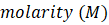. in other words it is defined as, the number of moles of a solute per litre of a solution is known as molarity. The molar concentration of a solution is another name for molarity. Molarity is temperature-dependent.

It varies inversely with temperature.

Molarity (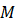)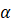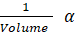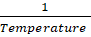Mathematically this term is expressed as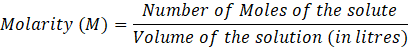For instance, if one mole of salt was dissolved in one litre of water, the resulting solution would not be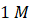. (read as "one molar"). The volume would be measured after the solution has been thoroughly mixed. Number of moles present in a solution divided by the total volume of the solution in litres, to determine the molarity.

## The quantity of a substance dissolved in a given mass of solvent is known as its molality (m), also known as molal concentration. In other words, it can also be defined as the moles of a solute dissolved in one kilogramme of a solvent. Molality is temperature independent.

Mathematically this term is expressed as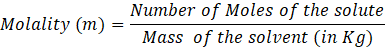For instance, if one mole of sugar was added to one litre of water, the resulting solution would not be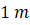. The mass would be measured after the solution has been thoroughly mixed. The number of moles of the solute dissolved is divided by the total mass of the solvent used for dissolving the solute to determine the molality.

## Molarity and Molality - Relationship

Both molarity and molality are very useful concentration expressions. They can also be related to the density of the solution for converting one to the other. The derivation of the relationship is given below.

Assume the given solute has a mass of W1 g =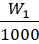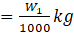.

The solute has a molar mass of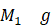.

The solvent is assumed to weigh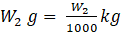.

Then, allow the volume of the prepared solution to be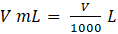.

We know that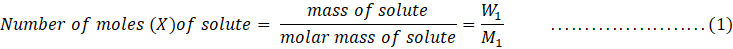According to the above formula molarity can be expressed asSimilarly,From equation (2) we get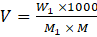……………(4)

From equation (3) we get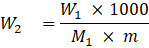Density,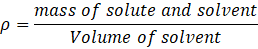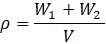Now,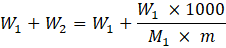Let’s multiply and divide by 1000 x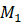on both numerator and denominator of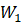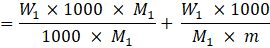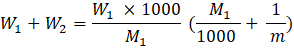Substituting the value of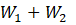and of V from equation (4) in the density formula we get,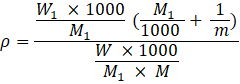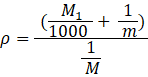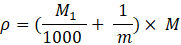Density of solution (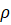)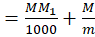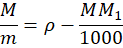The above equation gives the relationship between molarity and molality

## Practice Problems

Q1. Calculate the molality of the solution if the density of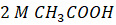the solution is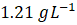.

1.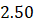m
2.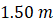3.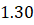m
4.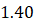m

Solution: We know, density of solution () =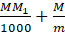Molar mass of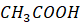=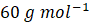=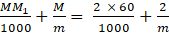= 0.12 +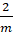= 1.21 - 0.12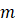=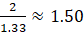Hence, correct option is (B).

Q2. Find molality of an aqueous solution of glucose having mole fraction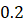and density of solution is 1.1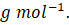1.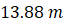2.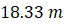3.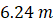4.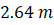Solution:

Molar mass of solute () = 180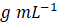Molar mass of solute (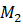) = 18Solution: We know, Mole fraction (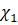) =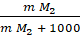=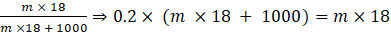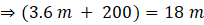Molality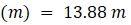Q3. Which of the following will change with an increase in temperature?

1. Molality
2. Molarity
3. Both of these
4. None of these

Solution

Molarity is temperature-dependent. it varies inversely with temperature.

mathematically: Molarity ()Q4. What is the unit of molarity?

1.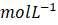2.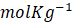3.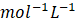4.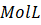Solution: The amount of a substance in a specific volume of solution is known as its. in other words it can also be defined as, The number of moles of a solute per litre of a solution is known as molarity. The molar concentration of a solution is another name for molarity.

Mathematically this term is expressed as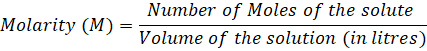Hence, the unit of molarity =Q1. Is it possible to convert any solution's molality into molarity without first knowing its density?

Answer: No, density is necessary for the conversion of molality into molarity or molarity into molality. While molality is only mass-dependent, molarity depends on volume as well.

The density of solution () =Q2. Can we consider an infinitely diluted solution's molarity to be equal to its molality?

Answer: Yes. We can take into account molarity = molality for an extremely diluted aqueous solution to maintain numerical simplicity.

Water has a known density of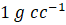. Thus, the weight of the solute becomes very small for very dilute solutions (negligible). The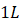volume of the solution now equals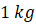. You can consider the weight of the solution to be the solvent's weight.

Q3. What distinguishes density from specific density?

Answer: Material-specific densities are calculated in relation to water. It is therefore absolute and unitless. density measured in grammes per litre or other units.

Q4. Does temperature have an impact on mole fraction?

Answer: No, the mole fraction is unaffected by temperature. We can determine the mole fraction of any component by measuring the masses of the solute and solvent.

Q5. How can the concentration of a solution be altered?

Answer: A worker might occasionally need to change the concentration of a solution by changing the amount of solvent. When a solvent is added to a solution, the concentration of solutes in the solution is diluted. Concentration involves the removal of solvent, which raises the concentration of solutes in the solution.

Q6. How do you make a solution with a known concentration?

Answer: It is possible to prepare solutions with known concentrations by either dissolving a known mass of the solvent solution and diluting it to the desired final volume or by diluting the acceptable volume of the more concentrated solution to the desired final volume (the stock solution).

Related Topics:Talk to our expert
Resend OTP Timer =
By submitting up, I agree to receive all the Whatsapp communication on my registered number and Aakash terms and conditions and privacy policy